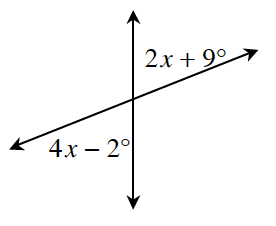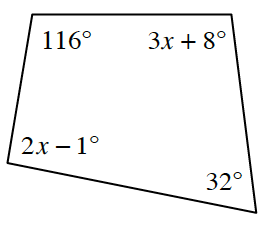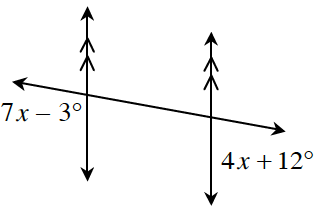### Home > CCG > Chapter 12 > Lesson 12.1.2 > Problem12-26

12-26.

For each relationship below, write and solve an equation for $x$. Justify your method.

1.How are these angles related?

$4x − 2^\circ = 2x + 9^\circ$
$11^\circ = 2x$
$x = 5.5^\circ$

1.What is the sum of the angles in a quadrilateral?

$x = 41^\circ$

1.How are these angles related?